# Round to Nearest 10, 100, or 1000 – Excel & Google Sheets

This tutorial will demonstrate how to round to the nearest 10, 100, or 1000 in Excel & Google Sheets.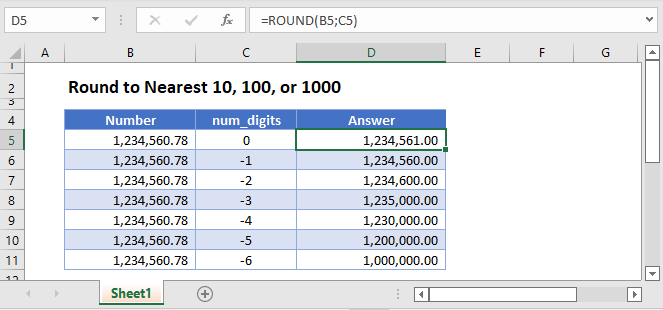## Round Function

The ROUND Function rounds a number to a specified number of digits relative to the decimal.

``=ROUND(B3,0)``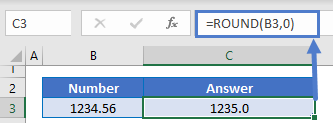By using negative numbers you can round to the nearest 10, 100, or 1000.

### Round to Nearest 10

``=ROUND(B3,-1)``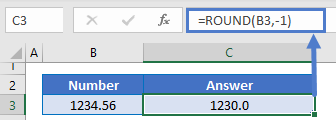### Round to Nearest 100

``=ROUND(B3,-2)``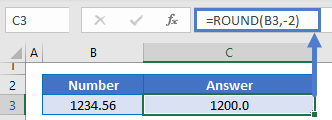### Round to Nearest 1000

``=ROUND(B3,-3)``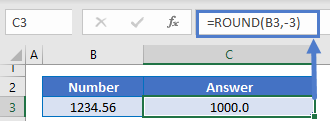## Round Up or Round Down to Nearest 100

You can use the ROUNDUP or ROUNDDOWN Functions to round a number up or down instead of using standard rounding rules. Here we will use these functions to round to the nearest 100.

### ROUNDUP Function

``=ROUNDUP(B3,-2)``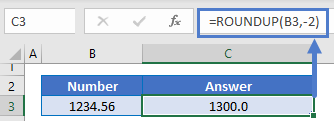### Easy Excel Automation### ROUNDDOWN Function

``=ROUNDDOWN(B3,-2)``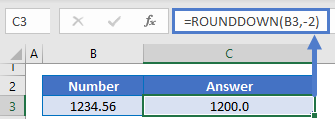## Round to Nearest 10, 100, or 1000 in Google Sheets

All of the above examples work exactly the same in Google Sheets as in Excel.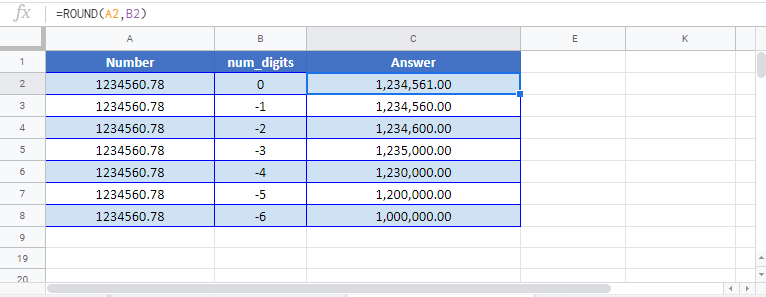### Excel Practice Worksheet

Practice Excel functions and formulas with our 100% free practice worksheets!